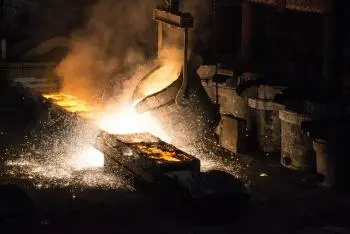# Heat Capacity: Definition, Formula, Types and ExamplesHeat capacity (also known as thermal capacity) is a property that indicates the total amount of heat that a substance or system can absorb or release when its temperature changes. It represents the capacity of an object or system to store thermal energy.

It is an extensive thermodynamics which means that its value depends on the amount of matter. In addition, its value depends on other properties such as the mass of the object or system, its chemical composition, its structure, and its ability to store thermal energy.

## Definition, What Is Heat Capacity?

The definition of heat capacity can be stated as the amount of heat required to raise the temperature of an object or system by one unit of temperature (usually degrees Celsius or Kelvin).

## Units

It is expressed in units of energy per degree Celsius or Kelvin, that is, as joules per degree Celsius (J/°C) or joules per Kelvin (J/K).

## Types of Heat Capacities

There are several types of heat capacities that are used to describe how the ability of an object or substance to store heat varies under different conditions. The most common types of heat capacities are:

1. Heat capacity at constant volume (Cv): It is the heat capacity of an object or substance when the volume is kept constant during the heat transfer process. In this case, there is no work done by the object or substance and all the transferred energy is converted into internal energy changes. Cv is used to describe how the temperature of a system changes at constant volume.

2. Heat capacity at constant pressure (Cp): It is the heat capacity of an object or substance when the pressure is kept constant during the heat transfer process. In this case, the object or substance can do work, such as expanding or compressing the system, as well as absorbing or releasing heat. Cp is used to describe how the temperature of a system changes at constant pressure.

## Heat Capacity Formula

The general formula for calculating the heat capacity (C) of an object or system can be expressed as follows:

C = Q / ΔT

Where:

• C is the heat capacity.

• Q is the amount of heat transferred or absorbed by the object or system.

• ΔT is the temperature change experienced by the object or system.

It is important to take into account that the heat capacity can vary depending on whether the process is carried out at constant volume (Cv) or at constant pressure (Cp).

### Molar Heat Capacity Formula

Also, in some cases, the molar heat capacity (Cm) is used, which represents the heat capacity per mole of substance.

The general formula for molar heat capacity is expressed as follows:

Cm = C/n

Where:

• Cm is the molar heat capacity.

• C is the heat capacity.

• n is the number of moles of substance.

## Importance of Heat Capacity: Use and Applications

This property is used in various practical applications. Here are some examples:

1. Design and optimization of heat transfer systems: it is essential to design and optimize heat exchangers, boilers, air conditioners and other refrigeration systems.

2. Prediction of temperature changes and thermal equilibrium: This property allows us to predict how the temperature of an object or system will change when heat is added to or removed from it.

3. Study of heating and cooling processes: In industrial, chemical and physical processes, it is essential to understand how materials and substances are heated or cooled under certain conditions.

4. Characterization of material properties: Heat capacity can be used to characterize the thermal properties of different materials, such as metals, liquids, gases, and solids.

5. Study of chemical reactions: In the field of chemistry, it is also important to understand and control chemical reactions since it allows calculating the amount of heat released or absorbed during the reaction.

## Examples of Heat Capacities

Here is a table showing examples of the heat capacity of different materials and substances at constant pressure.

 Substance Heat capacity (J/g°C) Description Water 4.18 Water has a high thermal capacity and is essential for thermal regulation on Earth. Aluminum 0.90 Aluminum is a lightweight metal commonly used in industrial applications. Iron 0.45 Iron is a metal frequently used in the manufacture of structures and machinery. Air 1.00 Dry air is a mixture of different gases present in the atmosphere. Lead 0.13 Lead is a heavy metal used in various industrial applications and batteries. Ethanol 2.44 Ethanol is a common alcohol and is used as a fuel and solvent.
Author:

Published: July 3, 2023
Last review: July 3, 2023